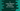# C program to swap adjacent elements of an one-dimensional array### C program to swap adjacent elements of a one-dimensional array :

In this tutorial, we will learn how to swap adjacent element of an integer array using C programming language. For example, if the array is [1,2,3,4,5,6], after swapping it will become [2,1,4,3,6,5]. The user will enter the elements of the array.Our program will swap the adjacent elements and then print out the final array. Let’s take a look at the program first :

### C program :

``````#include

int main(){
//1
int total;
int i;
int temp;

//2
printf("How many numbers you want to enter : ");
scanf("%d",&total);

//3
int array[total];

//4
for(i=0; i<total; i++){
printf("Enter element for position %d : ",i+1);
scanf("%d",&array[i]);
}

//5
printf("Array entered : \n");
for(i=0; i<total; i++){
printf("%d ",array[i]);
}

//6
for(i=0;i < total;i+=2)
{
//7
if(i+1 == total){
break;
}

//8
temp = array[i];
array[i]  = array[i+1];
array[i+1]= temp;
}

//9
printf("\nArray after reversing is done : \n");
for(i=0; i<total; i++){
printf("%d ",array[i]);
}

}``````

### Explanation :

1. Create one integer total to store the total number of elements for the array. Integer i to use in a loop and integer temp to store value temporarily while swapping elements.
2. Ask the user to enter how many numbers the array will contain. Store this value in variable total.
3. Create one integer array with the same length as the user entered.
4. Run one for loop and read all elements to store in the array. Read and store them.
5. Print the array that user has entered.
6. Run one more for loop to swap adjacent elements.
7. If the current value of i is pointing to the last element, break from the loop. This step helps us for odd sized array, because the last element is not required to swap in oddly sized arrays.
8. Swap element on position i and i + 1. Use the variable temp to hold one element temporarily while swapping.
9. After the swapping completed, print out the array elements again.

### Sample Output :

``````How many numbers you want to enter : 5
Enter element for position 1 : 1
Enter element for position 2 : 2
Enter element for position 3 : 3
Enter element for position 4 : 4
Enter element for position 5 : 5
Array entered :
1 2 3 4 5
Array after reversing is done :
2 1 4 3 5

How many numbers you want to enter : 6
Enter element for position 1 : 1
Enter element for position 2 : 2
Enter element for position 3 : 3
Enter element for position 4 : 4
Enter element for position 5 : 5
Enter element for position 6 : 6
Array entered :
1 2 3 4 5 6
Array after reversing is done :
2 1 4 3 6 5``````